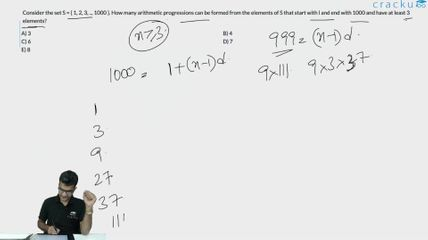Question 16

# Consider the set S = { 1, 2, 3, .., 1000 }. How many arithmetic progressions can be formed from the elements of S that start with l and end with 1000 and have at least 3 elements?

Solution

The nth term is a + (n-1)d
1000 = 1 + (n-1)d
So, (n-1)d = 999
999 = 3^3 * 37
So, the number of factors is 4*2 = 8
Since there should be at least 3 terms in the series, d cannot be 999.
So, the number of possibilities is 7

### View Video Solution